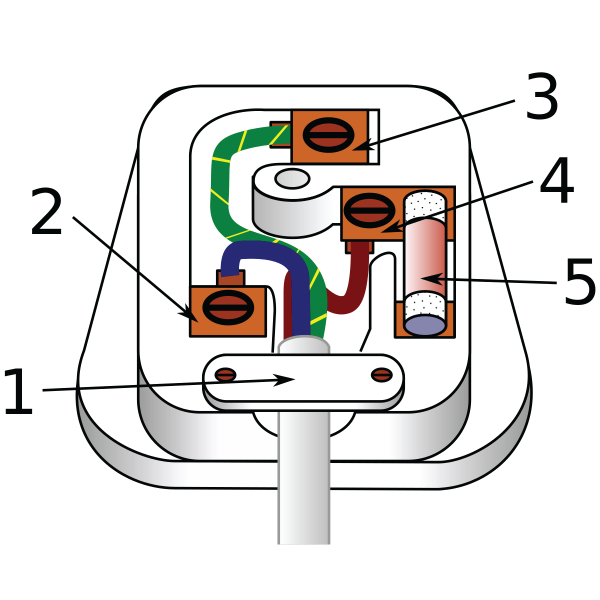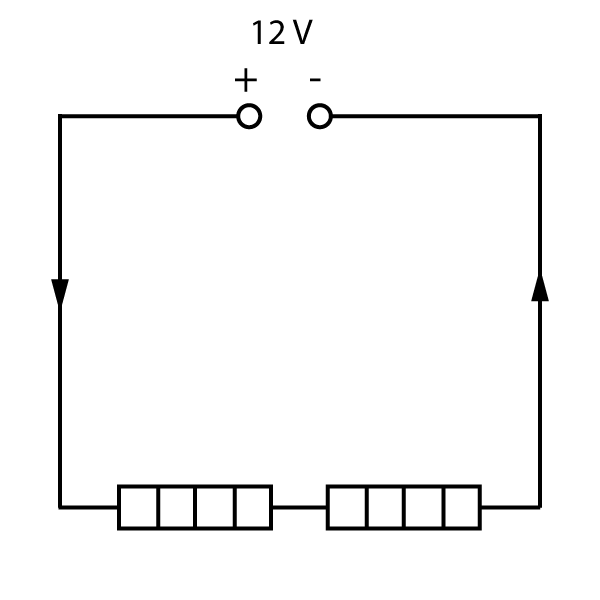TOPIC 2: ELECTRICITY

# 2.6 Mains electricity

If you have had the chance to carry out some experiments with cells and batteries at school, you will know that they are very safe to use. However the electrical sockets in homes, schools and offices are much more dangerous. The main reason for this is that the voltage is very high - typically 240 V in many countries. Japan and the USA use a lower 110 V to try to make it safer, but it is still very dangerous to humans and can deliver a potentially fatal electric shock.

### A.C./D.C.

Mains electricity is a.c., which stands for alternating current. The power station produces a current that constantly changes direction (a 50 Hz frequency in Europe). The power station that produces the electricity is effectively pushing and pulling electrons around the circuit. Note that an a.c. supply does not have a positive and negative terminal.
Cells, on the other hand, are d.c. - this stands for direct current, always flowing from positive to negative.DC supply symbolAC supply symbol

### Mains electrical plugs

The design and layout of plugs varies widely from country to country, but typically a plug has 2 or 3 'pins'. The picture below is a common internal layout with 3 pins.1 = cable grip
2 = neutral pin and wire (blue)
3 = earth pin and wire (green/yellow)
4 = live pin and wire (brown)
5 = fuse

Figure 1: A typical mains plug
(Wikimedia commons-Theresa Knott)

As this is an international course, you do not need to learn the layout of one particular country, but it is useful to understand some of the key parts of the plug:

• The live wire - this is the wire that is effectively connected to the power station, pushing and pulling current around the circuit. If you touch the live wire, you will receive a shock!
• The neutral wire - this is the 'return wire' completing the circuit back to the power station.
• The earth wire - this is the third wire that is frequently missing in some plugs, along with the third pin. It is an additional safety feature as we will learn about in the next section (2.7), but is not always needed.
• The cable grip - this simply locks the cable in place, preventing someone pulling on the connections, and possibly disconnecting part of the plug. Sparks inside the plug can cause fires.
• Fuse - this is another safety feature like the earth wire, that will be covered in the next section (2.7).

## Electrical power and energy

Mains sockets are used around our homes for devices that need a bit more power, for example cookers, hairdryers and lighting. Power (P) is a physical measurement covered in a bit more detail in topic 3. It is basically a measure of the energy delivered per second, and has units of the watt (W).
For electrical devices, the power delivered depends on two factors - the current flowing through it, but also the voltage at which this current is 'forced through' the device. A higher voltage and a higher current will lead to a very high power output.

To calculate electrical power, we use this formula:

power = current x voltage

P = I x V

[watts] = [amps] x [volts]

Learn this formula!

Note that in this formula, the voltage is actually the p.d. across the component, in cases where there are several devices connected in series to the supply.

In addition to this formula, we can generate a formula to find the energy transferred.
The energy transferred can be found by multiplying the power by the time over which the appliance is on. (See topic 3).

This gives the following new formula:

energy = current x voltage x time

E = I x V x t

[joules] = [amps] x [volts] x [seconds]

This formula will be provided for you in the exam, but learn what the letters mean!

Both these formulas are fairly straightforward, however watch out for power in kW (kilowatts), and time in minutes and hours. The formulas apply to both a.c. and d.c. voltages, and so can be used for questions with mains electricity and also with cells and batteries.

### Heating effect

Many of the devices we use at home rely on the heating effect of a current. When a current passes through any resistor, the power produced is thermal power, and this heat can be used for cooking, drying hair and heating rooms.

The standard symbol for a heater or heating element looks like a resistor with vertical lines (see the last section 2.5, and question 2 below). All heaters are basically resistors!

Take a look at the following example questions on this section - again try to do the question yourself before looking at the solution.

Questions:

1. A 240 V toaster uses a current of 2A.

• a) Calculate the power used by the toaster whilst in operation.
• b) When the toast pops up after 1 minute, the toaster turns off. Calculate the total energy transferred by the toaster.

a) We know that P = I x V, so:
P = 2 x 240
P = 480 W

b) Firstly, remember to use 60 seconds, not 1 minute.
For this part of the question we can use E = I x V x t
E = 2 x 240 x 60
E = 28 800 J (or 28.8 kJ)

2. The diagram below shows two heating elements connected in series. The current flowing through the elements is 2.5 amps.• a) Calculate the power output of 1 heating element.
• b) Calculate the total thermal energy produced from both elements over a 5 minute interval.

a) The p.d. across each element is 6 V, NOT 12 V.
Using P = I x V gives:
P = 2.5 x 6
P = 15 W each.

b) The total energy is given by E = I x V x t, and the total voltage is 12 V.
5 minutes = 300 seconds, so : E = 2.5 x 12 x 300
E = 9000 J (9.0 kJ)

3. A 230 V, 2kW mains kettle is used to boil a litre of water. This requires 340 kJ of energy.

• a) State one difference between mains electricity and the electricity from a 9V cell.
• b) Calculate the current through the heating element of the kettle.
• c) Calculate the time required to boil the water.

a) Mains is a.c., whereas a cell is a d.c. supply. (In addition, mains electricity is always much higher voltage than 9V, typically over 100 V worldwide).

b) We know P = I x V, so rearranging this gives:

 I = P V
 I = 2000 230

I = 8.70 amps

c) E = I x V x t can be rearranged to give:

 t = E I x V
 t = 340 000 = 340 000 8.7 x 230 2000

t = 170 seconds

(Note that we could have used the idea that E = P x t to solve part c, as the power is given in the question. If we use this method, then we get:

 t = E = 340 000 P 2000

t = 170 seconds)

Extension

Fancy a challenge?
This question is very hard, and is usually solved by learning a new formula at A level or IB physics level. However you can solve it without needing any other formulas apart from the ones covered so far in sections 2.1 to 2.4. Can you solve it?

Extension question:
A 60 mA current flows through a 1 kΩ resistor. What is the power lost as heat as current flows through the resistor?

The answer is close to 4 W but you will need a more accurate answer. If you get stuck on this, ask your teacher for help!

There are no additional questions for this section - move on to section 2.7 to learn about mains safety.

 MENU More.. Sign In HomeTopic 1: Forces Topic 2: Electricity Topic 3: Waves Topic 4: Energy Topic 5: Matter Topic 6: Magnetism Topic 7: Radioactivity Topic 8: Astrophysics
 1.1 Speed + Acceleration 1.2 Motion Graphs 1.3 Force + Vectors 1.4 Balancing Forces 1.5 Force Formulas 1.6 Stopping Distances 1.7 Hooke's Law Topic Quiz: